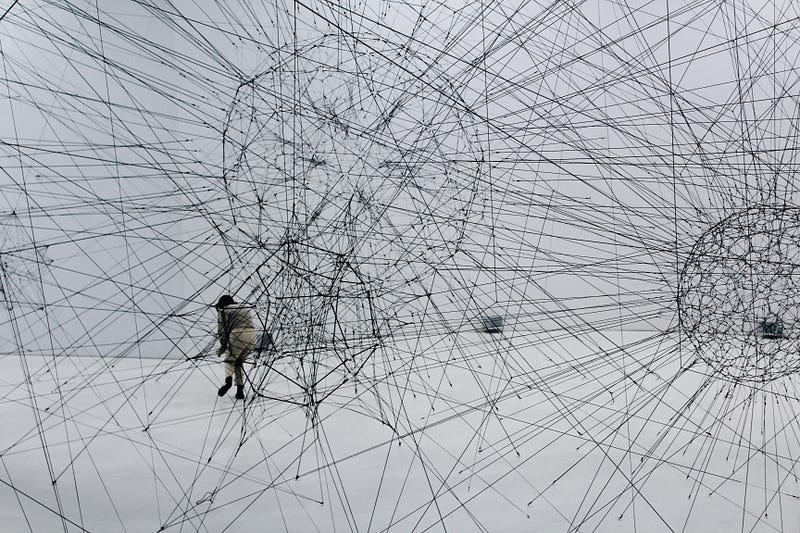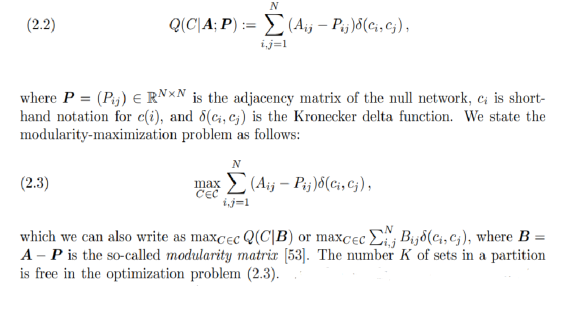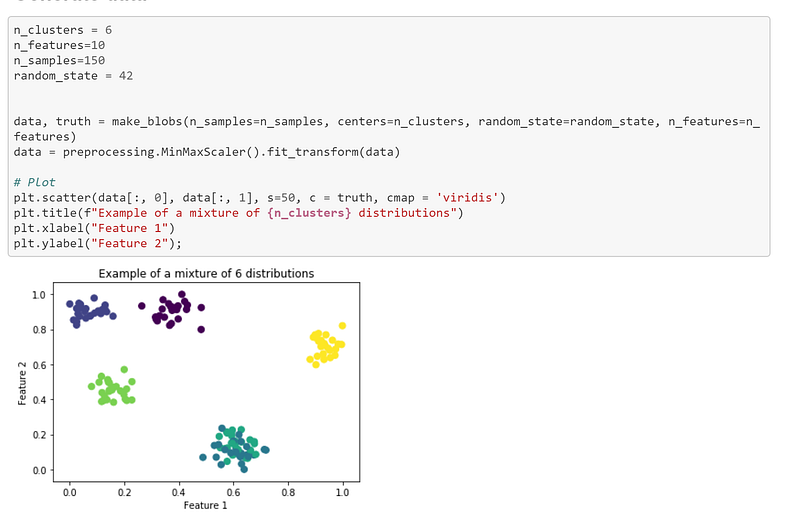Search
• Madalina Ciortan

# Clustering data with graph oriented techniques

## Introduction to Louvain graph community detectionThis post will show an alternative approach to clustering a dataset, which relies on graph oriented techniques, namely on louvain community detection.

## Louvain algorithm for community detection

### What are graph communities?

A community is a group of nodes within a network that are more densely connected than they are to the other nodes. The problem of community detection is the equivalent of partitioning a network into densely connected communities in which the nodes belonging to other communities are only sparsely connected. This achieved by optimizing a the modularity metric which evaluates the quality of a partitioning by how much more densely connected the nodes in a community are by how connected they would be on average, in a randomly defined network. The precise mathematical formulation is as follows:## Algorithm summary

In a nutshell the Louvain algorithm works in two steps: assignment of nodes to communities followed by redefining the network based on the communities found in the first phase, which become new coarse-grained nodes.

During the first step a node is selected at a time and put into the same community with its neighbor which would maximize the modularity function. Next another node is selected and the same heuristic is repeated until all nodes have been visited. The second step consists in merging each community into one course grained node and performing step one on this newly defined network. The process stops when it is not possible to improve the modularity any further. The complexity of this algorithm is n *log (n). Full details about this algorithm can be found in the original paper and also on this forum.

## Implementation

I made available a simple example running on simulated Gaussian 10 D clusters on Github.

We start by generating a less trivial test dataset, consisting of 6 clusters embedded in 10D:I developed two different approaches, one relying on embedding the input data set in a nearest neighbor graph and then using this very representation as input to the Louvain algorithm. The second approach starts from a distance matrix and uses the similarity between points as edge weights passed to the Louvain algorithm.

## Connectivity based approach

The first step is to obtain the connectivity matrix of the input data set and for this we will use Sklearn’s method kneighbors_graph, which computes the weighted graph of k-Neighbors points in the input data. This method can return either a connectivity matrix (binary matrix specifying if 2 vertices are connected or not which can be then used as input to the creation of the graph) or a distance matrix. We will chose the former option which provides a CSR sparse matrix. The non-zero elements in this matrix are edges in the graph.

I will use the igraph library to model the graph which is integrated with a louvain library providing multiple algorithms for optimizing the modularity.

```def cluster_by_connectivity(data, neighbors = 10, resolution_parameter = 1):
"""
This method partitions input data by applying the louvain algorithm
on the connectivity binary matrix returned by the kneighbors graph.
"""
A = kneighbors_graph(data, neighbors, mode='connectivity', include_self=True)
sources, targets = A.nonzero()
weights = A[sources, targets]
if isinstance(weights, np.matrix): # ravel data
weights = weights.A1
g = ig.Graph(directed=False)
g.add_vertices(A.shape)  # each observation is a node
edges = list(zip(sources, targets))
g.add_edges(edges)

g.es['weight'] = weights
weights = np.array(g.es["weight"]).astype(np.float64)
partition_type = louvain.RBConfigurationVertexPartition
partition_kwargs = {}
partition_kwargs["weights"] = weights
partition_kwargs["resolution_parameter"] = resolution_parameter
part = louvain.find_partition(g, partition_type, **partition_kwargs)
groups = np.array(part.membership)
return groups```

### Distance based approach

An alternative approach is to start from a distance matrix using for instance the euclidean distance between observations. Other distance measures can be considered, for instance correlation distance or cosine similarity, depending on the nature of the input data set. The distance matrix can be then transformed into a similarity matrix whose values can be considered as edge weights in the graph.

`distanceMatrix =  euclidean_distances(data, data)`

The full implementation is:

```def cluster_by_distance_matrix(distanceMatrix, resolution_parameter = 1.5):
"""
This method partitions input data by applying the louvain algorithm
on a given distance matrix.
A similarity matrix is computed from the distance matrix and its elements
will serve as edge weights.
"""
# convert distance matrix to similariy matrix
distanceMatrix = 1- distanceMatrix/np.max(distanceMatrix)
edges = np.unravel_index(np.arange(distanceMatrix.shape*distanceMatrix.shape), distanceMatrix.shape)
edges = list(zip(*edges))
weights = distanceMatrix.ravel()

g = ig.Graph(directed=False)
g.add_vertices(distanceMatrix.shape)  # each observation is a node
g.add_edges(edges)

g.es['weight'] = weights
weights = np.array(g.es["weight"]).astype(np.float64)
partition_type = louvain.RBConfigurationVertexPartition
partition_kwargs = {}
partition_kwargs["weights"] = weights
partition_kwargs["resolution_parameter"] = resolution_parameter
part = louvain.find_partition(g, partition_type, **partition_kwargs)
groups = np.array(part.membership)
return groups```

Both methods manage to retrieve correctly the input clusters. The second method needed an adaptation to the default resolution parameter, which is responsible for the following:

For larger networks, the Louvain method doesn’t stop with the “intuitive” communities. Instead, there’s a second pass through the community modification and coarse-graining stages, in which several of the intuitive communities are merged together. This is a general problem with modularity optimization algorithms; they have trouble detecting small communities in large networks. It’s a virtue of the Louvain method that something close to the intuitive community structure is available as an intermediate step in the process.

## References

https://arxiv.org/abs/0803.0476https://www.quora.com/Is-there-a-simple-explanation-of-the-Louvain-Method-of-community-detectionhttps://neo4j.com/docs/graph-algorithms/current/algorithms/louvain/#algorithms-louvain-context

See All